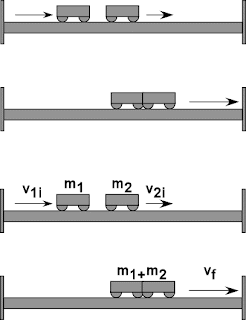## 2009-03-02

### Analysing Momentum

Momentum

The momentum of an object is the product of its mass and its velocity.

p = m X v

The principles of conservation of liner momentum states that the total linear momentum of a closed system is constant.

The linear momentum before and after a collision is conserved if there is no external force acting on it.

Elastic collision: linear momentum, kinetic energy and total energy are conserved.

Inelastic collision: only linear momentum and total energy are conserved and there is a loss in kinetic energy.

In an EXPLOSION, where two objects move in opposite directions, the total linear momentum before and after the explosion is zero.

The acceleration of a rocket leaving the earth increases because:
a) its mass is decreasing.
b) air resistance is decreasing.
c) gravitational pull is decreasing.

Conservation of Momentum

1. The term conservation is derived from the root word “conserve” which means constant.
2. The principle of conservation of momentum states that in the absence of an external force, the total momentum of a system remains unchanged.
3. An example of external force is friction and this can be contact friction or air friction.
4. An isolated or closed system the sum of external forces is zero, thus, the principle of conservation of momentum is true for a closed system.

Collisions

1. There are two types of collision:
(a) Elastic collision
(b) Inelastic collisions

2. In Elastic collision: Two objects collide and move apart again after a collision. Momentum is conserved. Total energy is conserved. Kinetic energy is conserved.
Formula: m1u1+m2u2 = m1v1+m2v2Elastic Collision

3. In Inelastic collision: Two objects combine and stop or move together with a same velocity after a collision. Momentum is conserved. Total energy is conserved. Kinetic energy is not conserved (the total kinetic energy after the collision is less than the total kinetic energy before collision, excess energy is released as heat, sound energy etc).
Formula: m1u1+m2u2 = (m1+m2)vInelastic Collision